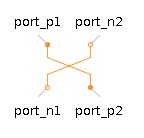Inverse Cosine - MapleSim Help

Inverse Cosine

Compute the arc cosineDescription The Inverse Cosine (or Acos) component computes the element-wise inverse cosine. The Signal Size parameter assigns the dimension of the input and output connectors.Equations ${y}_{j}=\mathrm{acos}\left({u}_{j}\right)$Connections

 Name Description Modelica ID $u$ Real input vector u $y$ Real output vector yParameters

 Name Default Units Description Modelica ID Signal Size $1$ Dimension of input and output signals signalSizeModelica Standard Library The component described in this topic is from the Modelica Standard Library. To view the original documentation, which includes author and copyright information, click here.